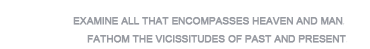•Publications
•Articles
•Books
•Representative articles
•Dessertations
•LinksTitle: Preliminary Research on the Mathematical Methods and Order of Problems in the “Fangcheng” Chapter of Yang Hui’s Mathematical Methods (1261 CE)
Author: Zhou Xiaohan
Jounal: Chinese Annals of History of Science and Technology
YEAR: 2020
Vol.: 4
No.: 1
Page: 1–62
Abstract:
Yang Hui was one of the most important authors of mathematical works during the thirteenth century. Mathematical Methods Explaining in Detail The Nine Chapters (Xiangjie jiuzhang suanfa 詳解九章算法, 1261 CE) is the earliest extant work attributed to Yang Hui. From the thirteenth to the fifteenth century, this work played a crucial role in the circulation and popularization of The Nine Chapters on Mathematical Procedures (Jiuzhang suanshu 九章算術). However, the only surviving printed edition of Mathematical Methods is incomplete and contains many mistakes obstructing contemporary researchers’ understanding of this work. The “Fangcheng” chapter of The Nine Chapters deals with problems related to solving what today are known as simultaneous sets of linear equations. However, interpreting the text in this chapter of Mathematical Methods and recovering the mathematical practices relating to fangcheng are difficult. Through detailed textual and mathematical analyses, the author of this paper explains Yang Hui’s understanding and practice relating to “the fangcheng method” and “the method of the positive and the negative.” This paper includes an appendix that provides a detailed translation of the ambiguous text relating to “the method of the positive and the negative” and gives reasons supporting the interpretation provided here. Yang Hui’s understanding of the concepts of “positive” and “negative” and his practice relating to these two concepts may easily be confused with their apparent counterparts in modern mathematics. Also, careful analysis of the mathematical methods in this work reveal that the order of problems in Yang Hui’s Reclassifications of Mathematical Methods Explaining in Detail The Nine Chapters ([Xiangjie jiuzhang suanfa zuanlei 詳解九章算法纂類], namely, the last section of Mathematical Methods) were rearranged according to commentaries to specific methods that appear in Mathematical Methods. Some textual clues referring to the “previous question” (qianwen 前問) in certain commentaries of Mathematical Methods indeed reflect the order of problems in Reclassifications. Yang Hui made especially detailed commentaries on the problems that he arranged in a sequence that differs with respect to the original order of problems as they appear in the ancient classic work, The Nine Chapters. All these discoveries reveal and serve to prove a close relationship between Yang Hui’s Mathematical Methods and his Reclassifications.